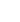15min:
UNCERTAINTIES IN PROPERTIES CALCULATED FROM FITTED POTENTIAL FUNCTIONS and DETERMINING POTENTIAL FUNCTIONS FROM FITS TO BOUND \to CONTINUUM INTENSITY DATA .

ROBERT J. LE ROY, Department of Chemistry, University of Waterloo, Waterloo, Ontario N2L 3G1, Canada.

In recent years it has become increasingly common to analyse spectroscopic data by using direct potential fits" of simulated data generated from analytic potential energy functions to experiment to optimize the parameters defining that potential energy function. This has the advantages of circumventing use of the semiclassical approximations associated with traditional methodologies, and of directly yielding a closed-form expression that is the most compact and comprehensive way to summarize what we know about a molecule. A question which then arises is: What are the uncertainties in properties computed using this potential? ~ It turns out that this question is very closely related to the question of how to apply this simulation/fitting method efficiently to the analysis of bound \to continuum intensity data. Both depend upon being able to answer the question: What are the partial derivatives of a wavefunction with respect to the parameters defining the potential energy function from which it is generated? ~ It will be shown that such partial derivatives may be obtained readily as the `particular' solutions of linear inhomogeneous differential equations of a type that is routinely solved in another context for calculating the centrifugal distortion constants of diatomic molecules., Applications of this technique have been incorporated into the publicly available bound-state data analysis and simulation program DPotFit and the distributed bound \to continuum simulation/fitting program BCONT.